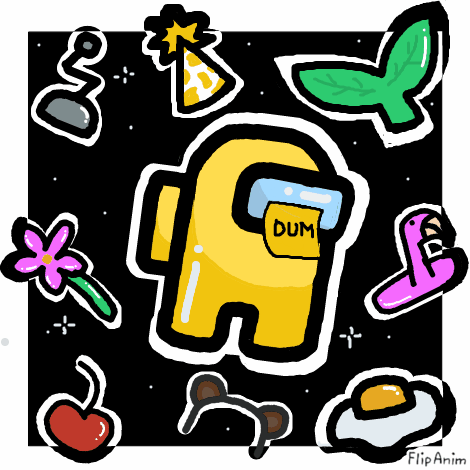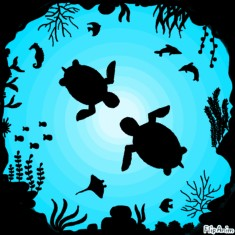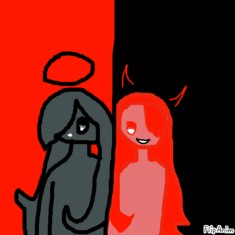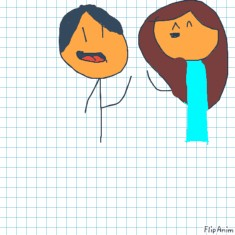- create flipbook animations online!
Among Us28.10.202028.10.2020 18:18
idk if i like this most of that hats look like poop but anyway its for this contest
Comment removed28.10.2020 20:47
How how how how how how how how how how how how how how how how how how how how how how how how how how how how how how how how how how how how how how how how how how how how how how how how how how how how how how how how how how how how how how how how how how how how how how how how how how how how how how03.11.2020 23:07
have you seen this girl named Pusheen101? I did she's so cool and cute! and love your anims!15.11.2020 01:16
I know you like avengers so look: https://www.youtube.com/watch?v=F_mhWxOjxp4